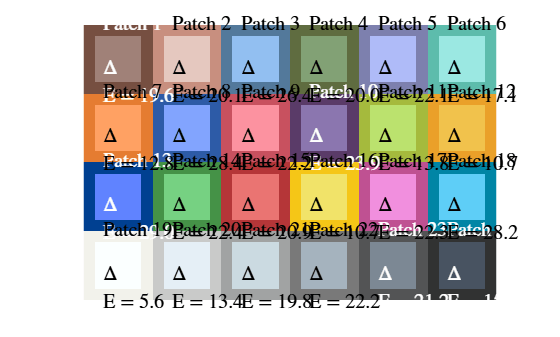Calculate CIE94 Color Difference of Colors on Test Chart

This example shows how to calculate the color difference of measured and reference colors using the CIE94 standard. By default, the measureColor function calculates the color difference between measured and reference colors on a test chart using the CIE76 standard. You can use the imcolordiff function to calculate the color difference using the CIE94 or CIEDE2000 standard.

Read an image of an X-Rite® ColorChecker® chart into the workspace.

Create a colorChecker object then display the chart with ROI annotations.

chart = colorChecker(I);
displayChart(chart)Measure the color in each color patch ROI and return the measurements in a table, colorTable. The color difference measurements in the Delta_E variable of the table follow the CIE76 standard.

colorTable = measureColor(chart);

On a color patch diagram, display the measured and reference colors with the corresponding CIE76 color difference superimposed on each patch.

displayColorPatch(colorTable)Extract the reference L*a*b* and measured RGB color values into a table.

referenceLab = colorTable{:,{'Reference_L','Reference_a','Reference_b'}};
measuredRGB = colorTable{:,{'Measured_R','Measured_G','Measured_B'}};

Convert the measured RGB colors to the L*a*b* color space, specifying a D50 white point.

measuredLab = rgb2lab(measuredRGB,"WhitePoint","d50");

Calculate the color difference using the imcolordiff function, specifying that the color measurements are in the L*a*b* color space. By default, this function calculates color differences using the CIE94 standard.

dE = imcolordiff(measuredLab,referenceLab,"isInputLab",true);

Create a new color table using the new color difference measurements.

colorTable94 = colorTable;
colorTable94{:,"Delta_E"} = dE;

On a color patch diagram, display the measured and reference colors with the corresponding CIE94 color difference superimposed on each patch.

displayColorPatch(colorTable94)